Sample Paper Chemistry Class 9

# Chemistry Unsolved Sample Paper Solutions ICSE Class 9

## Section-A

(i) (a) Salt and water

Explanation :

In a neutralisation reaction, acid and base reacts together to form salt and water.

(ii) (a) 1 : 8

Explanation :

Molecular mass of H2O is 18 out of which hydrogen is 1 × 2 = 2u and oxygen is 16u. The ratio is hence 2 : 16 or 1 : 8.

(iii) (b) Promoter

Explanation :

Substances which influence the rate of a chemical reaction by improving the efficiency of catalyst are called as promoters.

(iv) (d) Negative catalyst

Explanation :

Catalysts which retard the rate of reaction are called as negative catalysts.

(v) (c) Increases

Explanation :

For an endothermic process, solubility of a solute is directly proportional to the temperature. It increases with increase of temperature.

(vi) (a) Na2SO4. 10H2O
Explanation :

Glauber’s salt is chemically decahydrate sodium sulphate, also known as the mineral mirabilite. It is widely used by chemical industry. It’s formula is Na2SO4.10H2O.

(vii) (b) 2n2
Explanation :
The maximum possible number of electrons in a particular shell can be calculated using the formula 2n2, where n is the serial number of that shell.
(viii) (c) 6C14
Explanation :

Radiocarbon dating is also simply called carbon-14 dating. Carbon-14 is a radioactive isotope of carbon with a half-life of 5,730 years and decays into nitrogen. It is isotope which is used for determining the age of historical and geological material.

(ix) (c) Te

Explanation :

Along a period, the atomic radii of elements decreases on moving from left to right and down the group, atomic radii of elements increases.

(x) (c) Two

Explanation :

Alkaline earth metals are group 2 elements of the periodic table. Being in group 2, they all have 2 electrons in their valence shell.

(xi) (b) CO + H2
Explanation :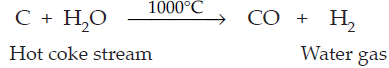(xii) (d) both (a) and (c)

Explanation :
In Bosch’s process, the removal of unreacted CO2 is done by dissolving mixture in water under pressure (30 atm.) or by dissolving mixture in caustic potash solution.

2KOH + CO2 → K2CO3 + H2O

(xiii) (d) All of the above

Explanation :

All the options listed are less polluting ones thereby decreasing the pollution and temperature which ultimately reduces the green house gas emission.

(xiv) (c) Using CFCs.

Explanation :

CFCs are atmospheric pollutants. They are main synthetic pollutants emerged in the beginning of 1930’s due to the refrigerators and cooling units which has now entered into the industrial sectors recently.

(xv) (c) 327°C

Explanation :• (i) The outermost shell of all the noble gases is complete. Thus, its valency is zero. ‘M’ has three electrons more than the noble gas. Thus, the valency of the element ‘M’ is +3. So,
• (a) MCl3
• (ii) M2(SO4)3
• (iii) M(OH)3
• (iv) MPO4
• (v) M2O3
(ii)
 Column A Column B (a) Cm3 1. Volume (b) Torr 2. Pressure (c) Charle’s law 3. V/T = V1/T1 (d) Kelvin 4. Temperature (e) Boyle’s Law 5. PV = P1V1
• (iii) (a) Hydrogen
• (b) Donor
• (c) Endothermic
• (d) Hydrogen sulphide
• (e) – 273ºC
(iv)
 Constituents of mixture Nature of mixture Examples (i) Solid-Solid Homogeneous Alloys (ii) Solid-Liquid Homogeneous Salt in water (iii) Gas-Gas Homogeneous Air (iv) Liquid-Liquid Homogeneous Milk in water (v) Gas-Solid Homogeneous Smoke
• (v) (a) 1. They are good conductors of electricity in the fused or aqueous state because electrostatic forces of attraction between ions in the solid state are very strong, and these forces weaken in the fused state or in the solution state. Hence, ions become mobile.
• 2. In electrovalent compounds, there exists a strong force of attraction between the oppositely charged ions, and a large amount of energy is required to break the strong bonding force between ions. So, they have high boiling and melting points. While in covalent compounds, weak forces of attraction exist between the binding molecules, thus less energy is required to break the force of binding. So, they have low boiling and melting points.
• (b) 1. As water is a polar compound, it decreases the electrostatic forces of attraction, resulting in free ions in aqueous solution. Hence, electrovalent compounds dissolve. While covalent compounds do not dissolve in water but dissolve in organic solvents. Organic solvents are non-polar; hence, these dissolve in non-polar covalent compounds.
• 2. Polar covalent compounds conduct electricity because they form ions in their solutions.
• 3. Electrovalent compounds are usually hard crystals yet brittle because they have strong electrostatic forces of attraction between their ions which cannot be separated easily.

## Section-B

• (i) Comparisons of Dalton’s atomic theory with the modern atomic theory. Dalton’s atomic theory :
• (a) Atoms are indivisible.
• (b) Atoms of the same element are similar in every respect.
• (c) Atoms combine in a simple whole number ratio to form molecules.
• (d) Atoms of different elements are different.
• (e) Atoms can neither be created nor be destroyed.
• Modern atomic theory :
• (a) Atoms are no longer indivisible and consist of electrons, protons, neutrons and even more subparticles.
• (b) Atoms of the same element may differ from one another called isotopes.
• (c) Atoms of different elements may be similar called isobars.
• (d) Atoms combine in a ratio which is not a simple whole number ratio; e.g., in sugar, the C12H22O11 ratio is not a whole number ratio.
• (ii) (a) 2 Fe + 3 Cl2 → 2 FeCl3
• (b) 2 KClO3 → 2 KCl + 3O2
• (iii) (a) Electronic configuration is the arrangement of electrons in the atomic or molecular orbitals of atoms or molecules.
• (b) Atomic number of elements is the number of protons in an atom of the element.
• (c) Atomic mass of an element is the total mass of protons and neutrons in one atom of the element.
• (iv) (a) Endothermic
• (b) Temperature
• (c) Water

(i) Temperature for the volume of a gas to be doubled initially at STP if the pressure is: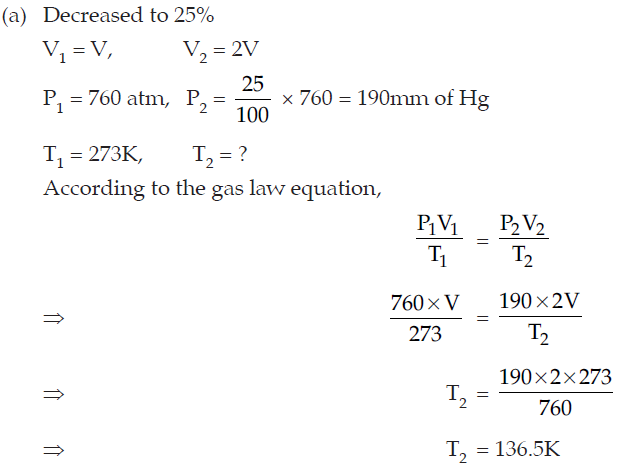(ii) Given, mass of empty dish = 52g
Mass of dish and solution is = 67g
Mass of solution = 67g – 52g = 15g
So, Mass of dish residue is = 54.3g
⇒ Mass of residue = 54.3g – 52g
= 2.3g

⇒ Mass of solvent = 15g – 2.3g
= 12.7g
12.7g of water dissolves = 2.30g of solid

100g of water dissolves =
2 .30 × 100 /127
= 18.11g

Hence, solubility of potassium nitrate is 18.11g.

• (iii) In this case, valency of M is +2 because metal M combine with two chlorine atoms having – 1 valency each. Thus,
• (a) MCO3
• (b) M(NO3)2
• (c) M(OH)2
• (iv) (a) A molecular formula represents a molecule.
• (b) Molecular formula of water (H2O) represents eighteen parts by mass of water.
• (c) A balanced equation obeys the law of conservation of mass, while an unbalanced equation does not obey this law.

• (i) (a) F < N < Be < Li
• (b) Cl < Br < I < At
• (ii) (a) Reduction reaction
• (b) Oxidation reaction
• (iii) (a) Zn + 2HCl → ZnCl2 + H2 is a Displacement reaction.
• (b) 4Na + O2 → 2Na2O is a Combination reaction.
• (c) NaCl + AgNO3 → AgCl + NaNO3 is a Double dispalcement reaction.
• (iv) (a) Ammonium hydroxide
• (b) Ammonium acetate
• (c) Soluble in hot water

• (i) (a) If ozone gas is present in atmosphere, then living organisms cannot survive because it is highly poisonous gas.
• (b) We will not be able to survive on Earth, if all plants become extinct from the earth because plants are the only source of oxygen in the atmosphere.
• (ii) Weight of water in solution = 12g – 3g = 9g
• 9g of water dissolves = 3g of solid
• Therefore, 100g of water will dissolve=3x100/9= 33.3g
• Thus, solubility of KCl in water at 20°C is 33.3g
• (iii) (a) Orbital structure of HCl: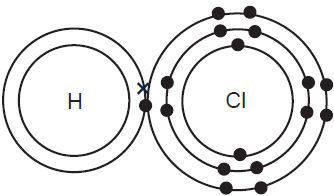• (b) Orbital structure of Cl2: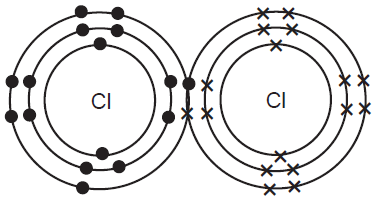• (c) Orbital structure of Hydrogen Sulphide (H2S):• (iv) (a) Double displacement reaction.
• (b) Displacement reaction.
• (c) Combination reaction.

• (i) (a) Relative molecular mass: Relative molecular mass of a molecule is the average molecular mass of substance when compared with 1/12 of the mass of an atom of Carbon-12.
• Relative molecular mass of a molecule
• =Average molecular mass of one molecule of a substance/Mass of 1/12 of an atom of Carbon-12
• (b) Molecular weight of Na2CO3.10H2O = 23 × 2 + 12 + 16 × 3 + 20 × 1 + 10 × 16
• = 46 + 12 + 48 + 20 + 160
• = 286u
• (ii) (a) A solubility curve is a line graph that plots changes in solubility of a solute in a given solvent against changing temperature.
• (b) Applications of solubility curve :
• 1. Shape of the curve indicates how the solubility of the given substance in a solvent varies with change in temperature. The solubility of a substance at a particular temperature can be determined from the curve.
• 2. The effect of cooling of hot solutions of different substances can be found from the curves.
• (iii) (a) A – Nitrogen
• B – Hydrogen
• C – Ammonia
• (b) Gas ‘C’ is manufactured by the Haber’s process.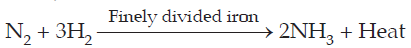• Favourable conditions:
• 1. Temperature should be between 450°C and 500°C.
• 2. Pressure should be high (200–1000 atm.).
• 3. Promoter used should be molybdenum.
• (c) 1. It turns moist red litmus blue.
• 2. NH3 + HCl → NH4Cl
•            (Conc)   (Dense white fumes)
• When gas ‘C’ comes in contact with conc. HCl, Ammonium chloride produced with dense white fumes.
• (iv) (a) Three volumes of hydrogen and one volume of nitrogen reacts at temperature 450–500°C and pressure 200–900 atm in the presence of finely divided iron catalyst with molybdenum as promoter to give ammonia.• (b) Equal volumes of hydrogen and chlorine react slowly in diffused sunlight to form hydrogen chloride.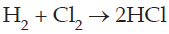• (c) Hydrogen gas on passing through molten sulphur reacts to give hydrogen sulphides.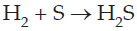• (i) (a) Number of proton is equal to atomic number. So, number of protons in nitrogen atom is 7.
• (b) In an atom, number of proton and number of electron is equal. So, number of electrons in nitrogen atom is 7.
• (c) Mass number = Number of proton + Number of neutron
• Number of neutron = 14 – 7 = 7
• Hence, number of neutrons in nitrogen atom is 7.
• (d) Electronic configuration of nitrogen atom is 2, 5. As the valence shell consist of five electrons it means, it accept electrons from other element to complete his octate and make electronegative element which is a unique property of non-metals. So, nitrogen is a non-metal. So, complete table is,
 Element Mass number Atomic number Number of protons Number of electrons Number of neutrons Metal or non-metal Nitrogen 14 7 7 7 7 non-metal
• (ii) (a) Difference between molecular mass and empirical formula mass.
 Molecular mass Empirical formula mass It is the sum of atomic masses of the constituent atoms present in one molecule of that substance. For example: Molecular mass of C2H2 is 26 u. It is simplest whole number ratio of each type of atom in a compound and the sum of atomic masses of corresponding elements present in the empirical formula. For example: C2H2, its empirical formula is CH. So, empirical formula mass is 13 u.
• (b) Difference between metals and non-metals.
 Metals Non-metals They are elements with one, two or three electrons in their outermost shell. They are elements with five, six or seven electrons in their outermost shell.
• (iii)
 Formula of radicals Valency (i) Sulphate SO42– –2 (ii) Sulphite SO32– –2 (iii) Sulphide S2– –2
• (iv) (a) A solid non-metal of valency 3 has atomic number 15.
• (b) A gas of valency 2 has atomic number 8.
• (c) A metal of valency 1 has atomic number 19.

#### ICSE 61 Sample Question Papers

All Subjects Combined for Class 9 Exam 2023

The dot mark field are mandatory, So please fill them in carefully
To download the Sample Paper (PDF File), Please fill & submit the form below.

Free Shipping on all
orderes over ₹ 1,000

Free 10-Day
Return Policy

15-Days Money-back
Guarantee

23,125 Products
Shipped this Month

Need Help?
+91-745 507 7222

Shopping cart